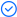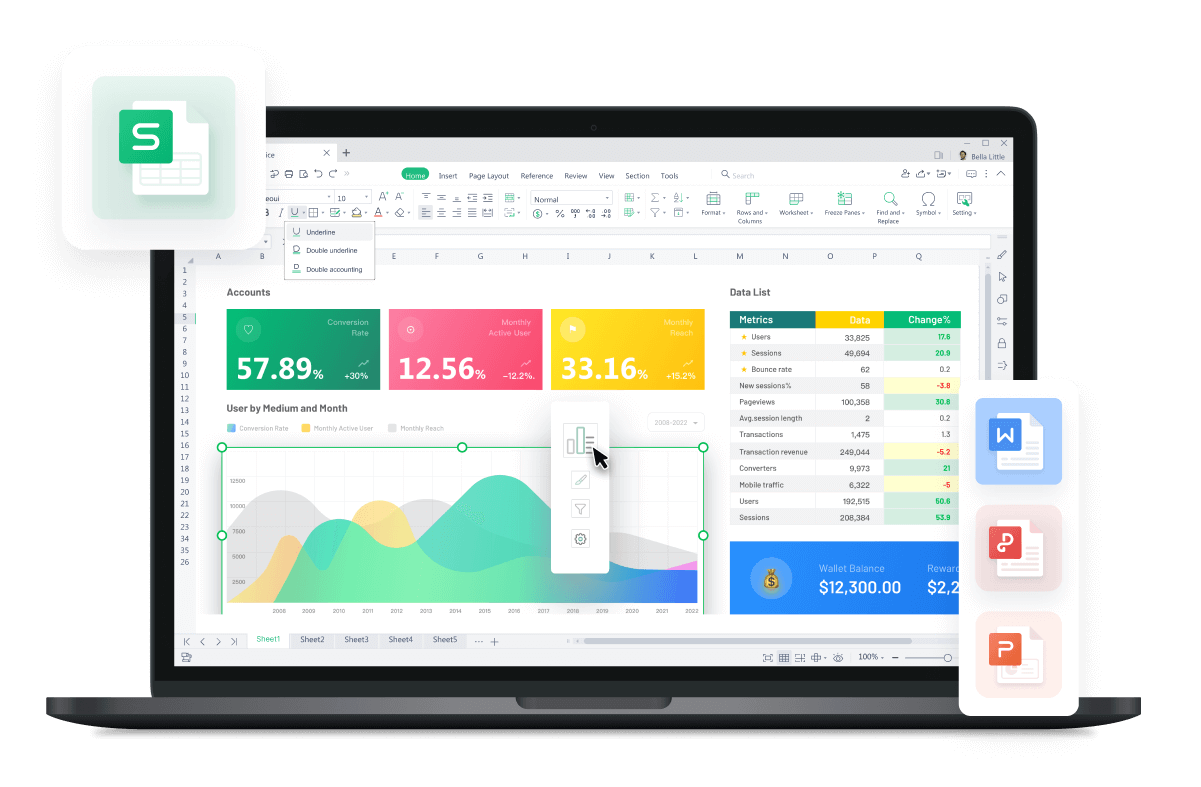WPS Office

Free All-in-One Office Suite with PDF EditorRead, edit, and convert PDFs with the powerful PDF toolkit.Microsoft-like interface, easy to use.

Windows • MacOS • Linux • iOS • Android# How to use if function with text in excel

August 1, 2022
8.0K Views

There are many powerful functions within Microsoft Excel, some of which are less well known than others. One such function is the IF function, which is designed to take a value or set of values and then return a result or set of results based on those values. The IF function will then take this value and test it against another value to see what should be returned as a result. I

F Function in Excel can also be used to return text output or several types of outputs like text, number, date, and logical values? Let us explore the IF Function in detail then! In this tutorial, we'll be looking at how to use the IF function with text in Excel. This straightforward but effective way to get your data into a spreadsheet for quick analysis.

Tips that are provided in this article are compatible with versions 2010/2013/2016.

How To Use If Function With Text In Excel: Finding Specific Text

If you need to find a specific piece of text in one or more cells, you can easily do so with the IF function.

1.For example, if you need to see if a specific word is contained in a cell or range of cells, you could use the following formula:

This formula would return Found! if A3 contains the word Hello, and Not Found otherwise.

Now you know how to use if function with text in Excel to check if a specific text exists or not.

How To Use If Function With Text In Excel: Finding Exact Text

If you are looking for an exact text match in Excel, then you can combine the IF and EXACT functions to get the desired output

1.Here is a sample formula to show how this can be done:

=IF(EXACT(A2,abc),1,0)

2.This formula will return 1 if there is an exact match and will return 0 in case of no match.

3.As you can see that there is no exact match, so it has returned us 0.

Now you know how to use if function to find exact text in excel.

How To Use IF Function In Excel: Finding Blank Cell

IF function can be combined with the  ISBLANK function to find if some text exists in a specific cell or not. This is useful when you want to check if a cell has any data in it, but you don't want to display any text if it doesn't. For example, if you have a list of products and want to show 'Discontinued' when there are no products available, then you can use this formula:

=IF(ISBLANK(B5),' Discontinued', “Available”)

Now you know how to use the IF function to check for blank cells in excel.

Did you learn how to use the IF function with text in Excel? You can follow WPS academy to learn more features of Word documents, Excel Spreadsheets, and PowerPoint slides.